# How to Solve a FIND THE FACTORS Puzzle

## Written explanation of how to solve

FIND THE FACTORS is a logic puzzle based on the multiplication table. In this table, the numbers 1 – 12 in the top row and first column are factors of the products listed on the inside of the table.Since several of the products are listed more than once, the chart below can be very helpful. It shows every product in the multiplication table and the factors from the table that make those products. (Since multiplication is commutative, 3 x 5 = 15 and 5 x 3 = 15. To save space both facts are simply listed as 5 x 3 with the greater factor listed first.)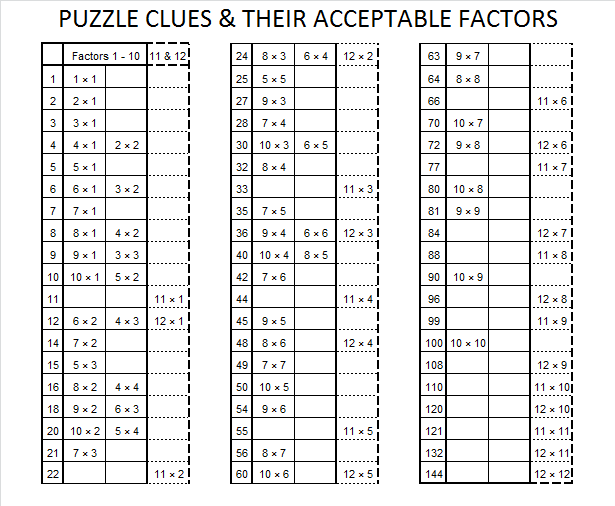Most people can fill out a multiplication table even if it looks like this:FIND THE FACTORS challenges you to complete a similar looking multiplication table except NONE of the factors are given! Only a few of the products are given as clues. To help you solve the puzzles, here are step by step instructions to solve a level 5 puzzle that includes most types of situations you may encounter trying to solve any of the puzzles

1. FIND THE FACTORS 1–10 is a logic puzzle. The object is to write each of the numbers 1 – 10 in both the top row (the factor row) and the first column (the factor column) so that those written numbers are the factors of the given clues. There is only one solution. Here is a sample puzzle: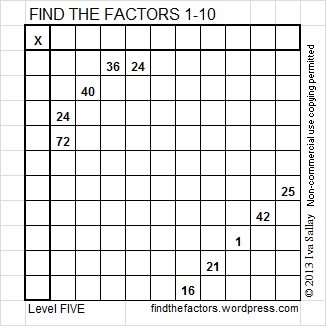—————————————————————————————————————

2. First look for the following perfect squares: 1, 25, 49, 64, 81, or 100 because there is only one way to factor each of those numbers so that both factors are not greater than ten. In this puzzle, the 1 must be 1 x 1 and the 25 must be 5 x 5. Note that perfect squares 16 and 36 both have more than one way to factor them using only factors less than 10, so deal with those clues later. (If you were trying to solve FIND THE FACTORS 1 – 12, you would also look for perfect squares 121 and 144.)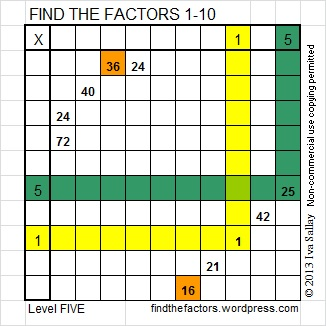—————————————————————————————————————-

3. Next look for a row or a column with at least 2 clues so that you can find a factor for both clues. This puzzle has one column and one row that qualify. However, the clues, 36 & 24, are not helpful yet because 2 different numbers (4 and 6) will factor into both 36 & 24. On the other hand, the column containing 24 and 72 is very useful because 8 × 3 = 24 and 8 × 9 = 72, and all of those factors are not greater than 10. Since 8 is the ONLY common factor meeting that condition, write 8 above that column and write 3 and 9 in the corresponding cells.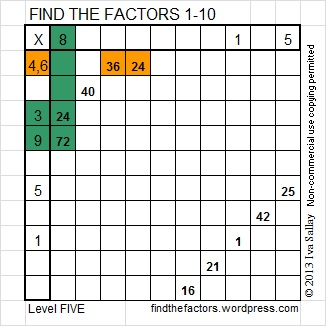—————————————————————————————————————-

4. Since you have found the 3 in the factor column, try to find the 3 in the factor row. Because the only way to factor 21 is 3 × 7, that has to be where the other 3 belongs. Likewise, since 42 = 7 × 6, you know where to write its factors. Write the 3, 7, 7, and 6 in the corresponding cells.—————————————————————————————————————-

5. Remember when we noticed that both 4 and 6 were factors of both 36 and 24? Finding that 6 in the factor column for the previous step means that 6 cannot also be the factor of the clue pair 36 & 24. Therefore, you have found where the 4 should be written in the factor column.  Write 4, 9, & 6 in their corresponding factor cells.—————————————————————————————————————-

6.  You know that a 2 must appear somewhere in the factor column so let’s consider everyplace 2 might go:

2 × 20 = 40, but you can’t write 20 in the factor row.

2 × 8 = 16, but there’s already an 8 in the factor row.

The only place in the factor column that the 2 can be written is in the cell next to the row with no clues.—————————————————————————————————————-

7.  The factors of 16 are 4 × 4 and 2 × 8. Since 4 already appears in either the factor row or the factor column, 4 × 4 cannot be the factors of 16 needed here.  Write 2 in the factor row and 8 in the factor column.—————————————————————————————————————-

8.  The only number 1 – 10 missing in the factor column is 10.  Since 10 × 4 = 40, write 10 & 4 in the cells.  Now count from 1 to 10 while you determine what number is missing in the factor row. In this case the missing number is 10.—————————————————————————————————————-

9.  Now all the factors have been found! At this point I don’t always fill in the rest of the table, but when I do, I can complete a 10 x 10 table in less than 3 minutes and a 12 x 12 table in less than 5. It would benefit any child to see an adult filling in a table just for the fun of it. What a powerful example of lifelong learning would be set!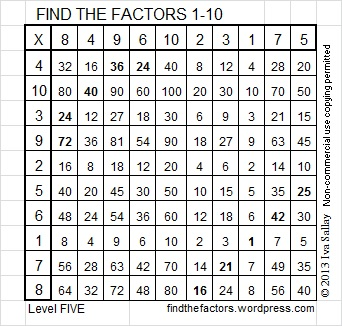All of the steps listed above can be summarized in a single chart.## Video explanations of how to solve:

Here I’ve made videos of each level of the Find the Factors 1 – 10 puzzles getting solved. My goal was to make the videos short, so each video is no more than 2 minutes 20 seconds long. Enjoy!

### An extra complicated level 6:

Even though every row and every column of this puzzle has at least one clue, the logic is very complicated. I’ve written several notes on the puzzle, but it will still take a couple of minutes just to use logic to figure out where to start.

### Challenge Puzzle:

I explain how to use logic to get started on this Find the Factors 1-12 Challenge Puzzle:

## 13 thoughts on “How to Solve a FIND THE FACTORS Puzzle”

1.Paula Beardell Krieg

Okay, that was easier to understand that I thought it would be. It’s 6:30pm, my husband is busy clearing off snow from the driveway, the puppy has found a comfy nook, and I am going to try my hand at puzzle 321.

2.Demetrio Colmenarez

I’m starting a blog on Mathematics in spanish, and I want to know if if you could give me permission
to use some images from your blog. Many thanks.

•ivasallay

Thank you for your interest. I’m assuming you would like to use just a few of my images. If the image has findthefactors.com on it, you may use it. For any other images, you can probably use them, but I will decide on a case by case basis and you would have to state they are from findthefactor.com. Good luck on your new blog!

3.Małgorzata

THANK YOU!

•ivasallay

You’re welcome!

4.naod

hi there,
I was just wondering how you would solved the skipoku?

•ivasallay

All of the clues in this Skipokuare multiples of 7. You solve it just like a sudoku puzzle but instead of counting from 1 to 9 over and over again, you skip count from 7 to 63 repeatedly.

This site uses Akismet to reduce spam. Learn how your comment data is processed.